Case Based Questions (MCQ)

Chapter 1 Class 10 Real Numbers
Serial order wise

## To enhance the reading skills of grade X students, the school nominates you and two of your friends to set up a class library. There are two sections- section A and section B of grade X. There are 32 students in section A and 36 students in section B.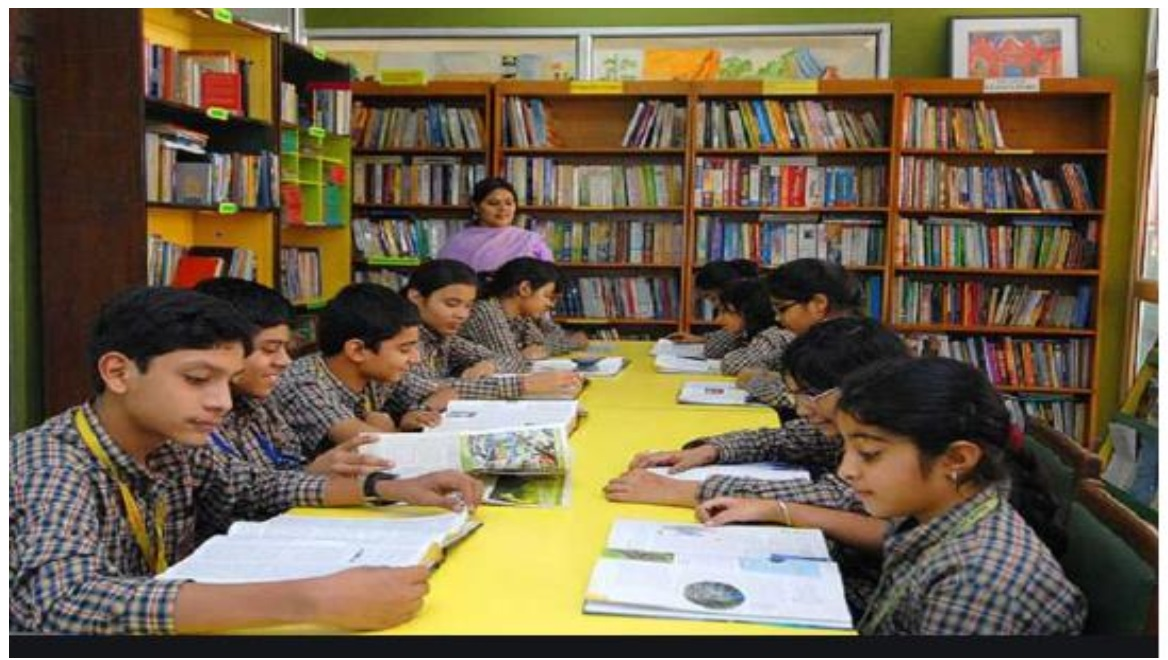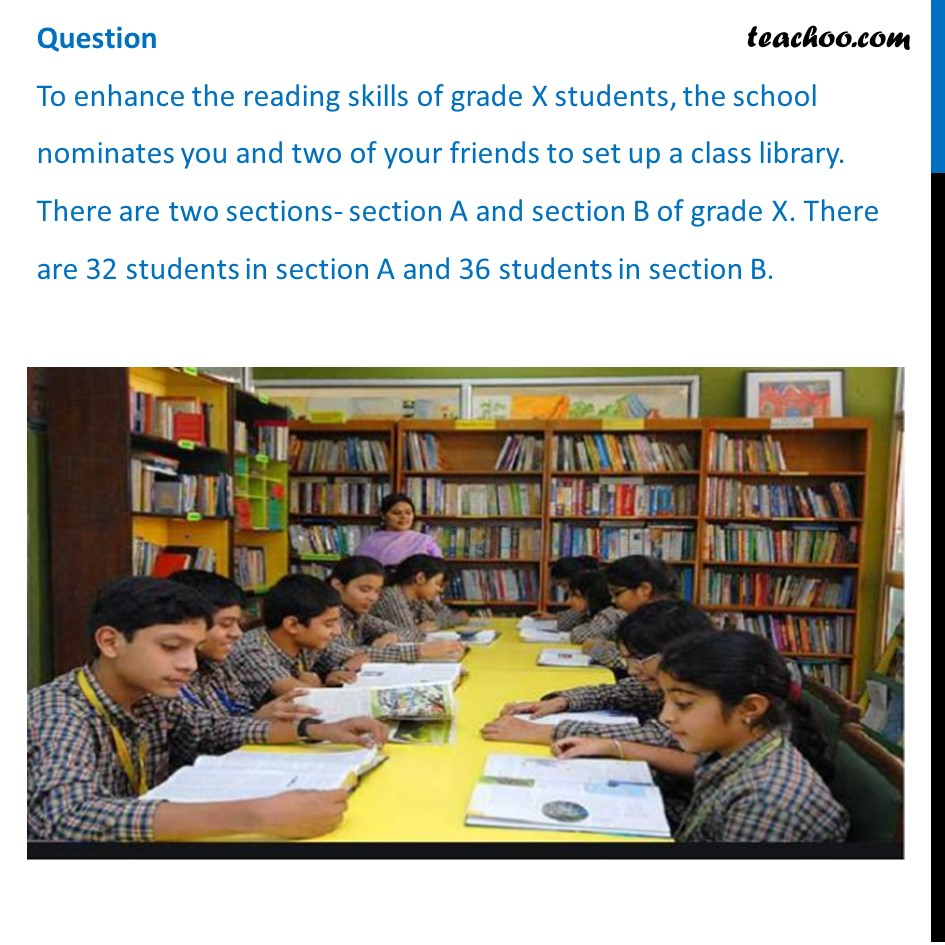## What is the minimum number of books you will acquire for the class library, so that they can be distributed equally among students of Section A or Section B? (a) 144   (b) 128   (c) 288  (d) 272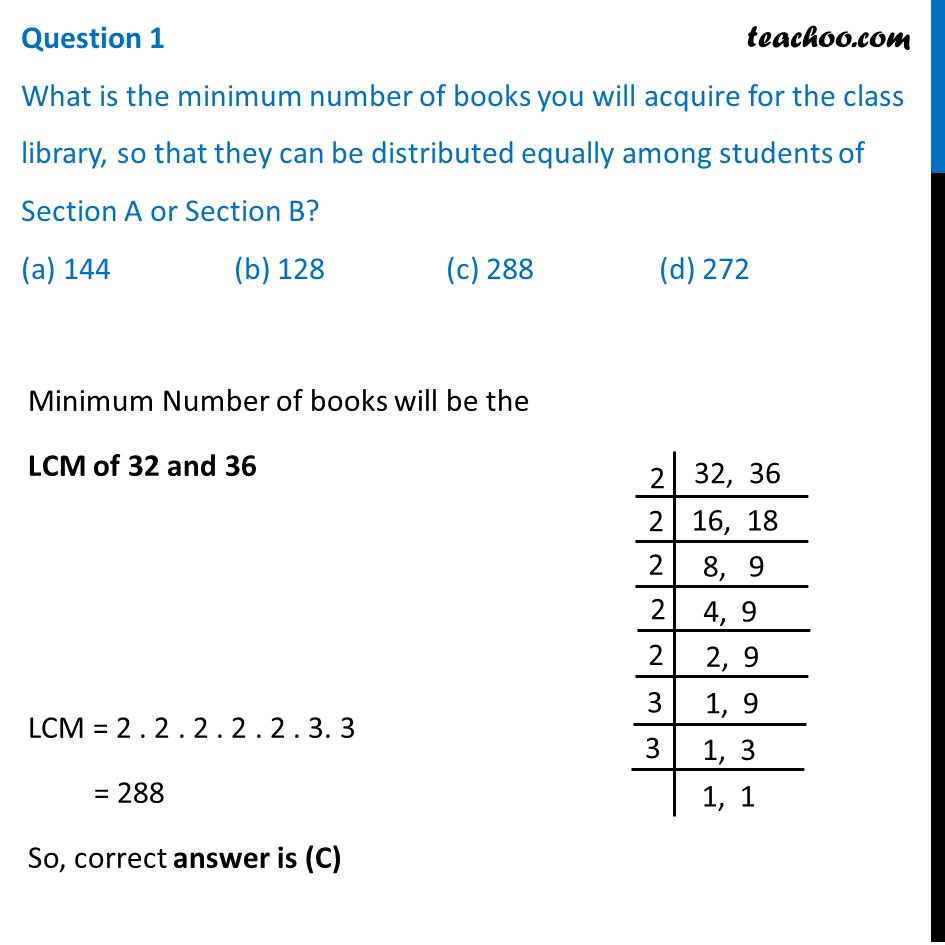## If the product of two positive integers is equal to the product of their HCF and LCM is true then, the HCF (32 , 36) is (a) 2  (b) 4   (c) 6  (d) 8## 36 can be expressed as a product of it’s primes as (a) 2 2   × 3 2 (b) 2 1 × 3 3 (c) 2 3 × 3 1 (d) 2 0 × 3 0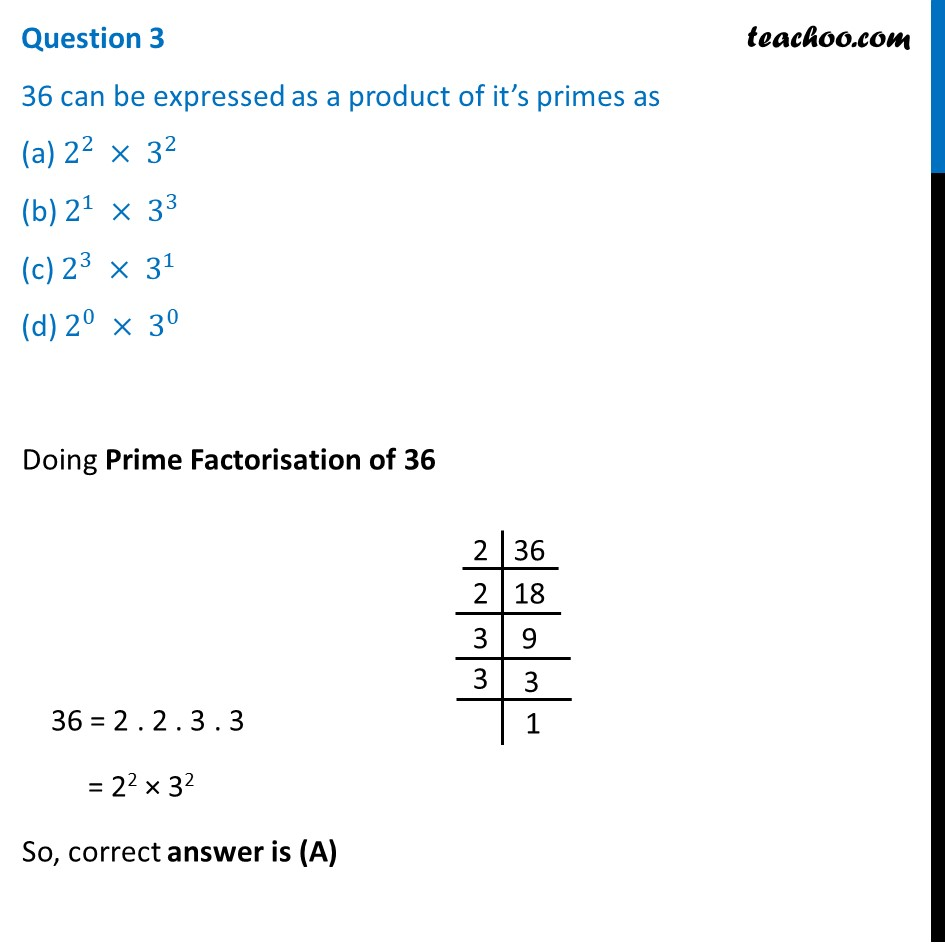## 7 × 11 × 13 × 15 + 15 is a (a) Prime number  (b) Composite Number  (c) Neither prime nor composite  (d) None of the above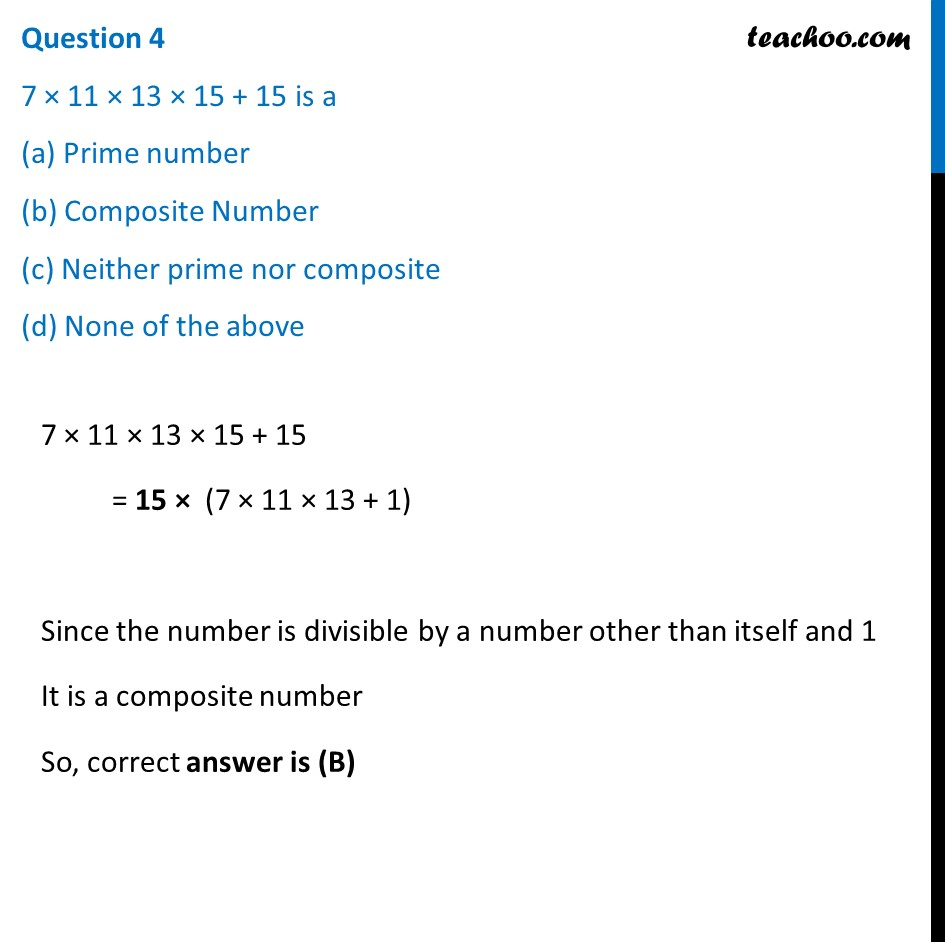## If p and q are positive integers such that p = ab 2 and q = a 2 b, where a, b are prime numbers, then the LCM (p, q) is (a) ab  (b) a 2 b 2 (c) a 3 b 2 (d) a 3 b 3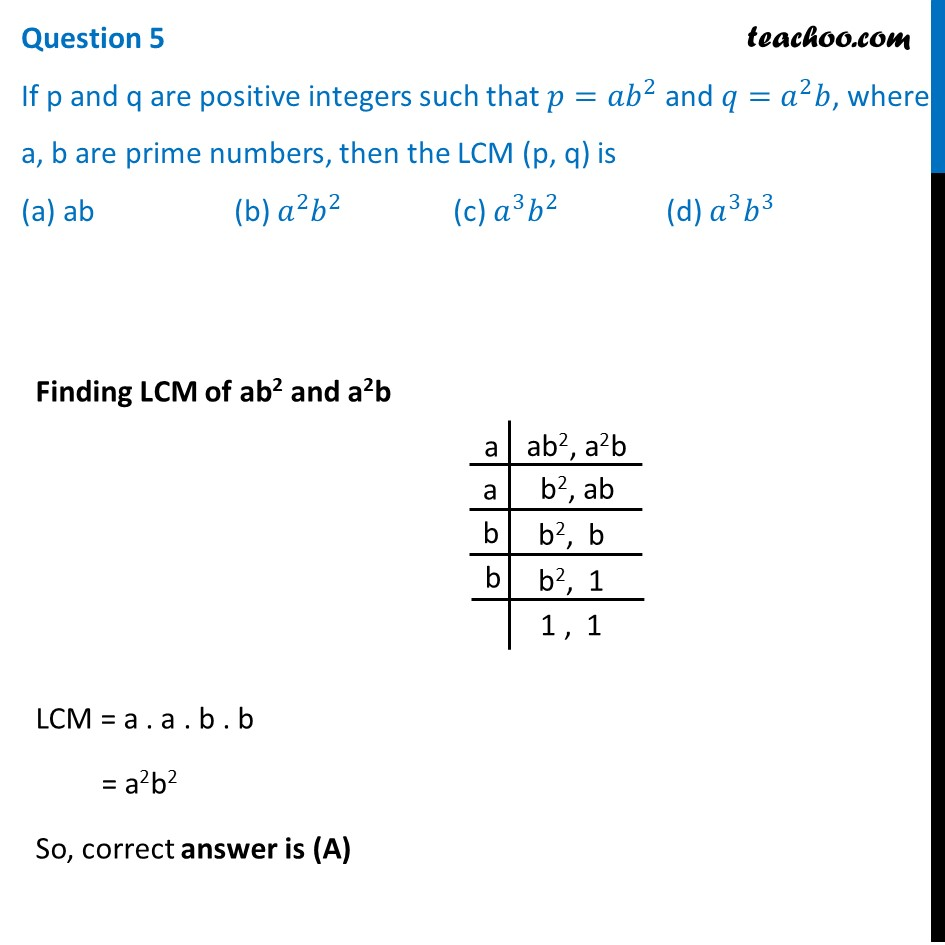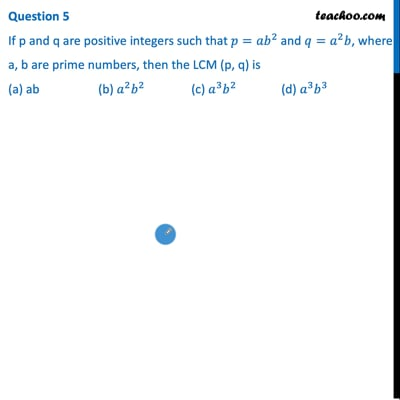This video is only available for Teachoo black users

Solve all your doubts with Teachoo Black (new monthly pack available now!)

### Transcript

Question To enhance the reading skills of grade X students, the school nominates you and two of your friends to set up a class library. There are two sections- section A and section B of grade X. There are 32 students in section A and 36 students in section B.Question 1 What is the minimum number of books you will acquire for the class library, so that they can be distributed equally among students of Section A or Section B? (a) 144 (b) 128 (c) 288 (d) 272 Minimum Number of books will be the LCM of 32 and 36 LCM = 2 . 2 . 2 . 2 . 2 . 3. 3 = 288 So, correct answer is (C) Question 2 If the product of two positive integers is equal to the product of their HCF and LCM is true then, the HCF (32 , 36) is (a) 2 (b) 4 (c) 6 (d) 8 Given that Product of two numbers = HCF × LCM Putting two numbers as 32, 36 and their LCM as 288 32 × 36 = HCF × 288 (32 × 36)/288 = HCF HCF = (32 × 36)/288 HCF = 36/9 = 4 So, correct answer is (B) Question 3 36 can be expressed as a product of it’s primes as (a) 2^2 × 3^2 (b) 2^1 × 3^3 (c) 2^3 × 3^1 (d) 2^0 × 3^0 Doing Prime Factorisation of 36 36 = 2 . 2 . 3 . 3 = 22 × 32 So, correct answer is (A) Question 4 7 × 11 × 13 × 15 + 15 is a (a) Prime number (b) Composite Number (c) Neither prime nor composite (d) None of the above 7 × 11 × 13 × 15 + 15 = 15 × (7 × 11 × 13 + 1) Since the number is divisible by a number other than itself and 1 It is a composite number So, correct answer is (B) Question 5 If p and q are positive integers such that 〖𝑝=𝑎𝑏〗^2 and 𝑞=𝑎^2 𝑏, where a, b are prime numbers, then the LCM (p, q) is (a) ab (b) 𝑎^2 𝑏^2 (c) 𝑎^3 𝑏^2 (d) 𝑎^3 𝑏^3 Finding LCM of ab2 and a2b LCM = a . a . b . b = a2b2 So, correct answer is (A)